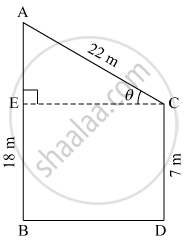Share

# Two Poles of Heights 18 Metre and 7 Metre Are Erected on a Ground. the Length of the Wire Fastened at Their Tops in 22 Metre. Find the Angle Made by the Wire with the Horizontal. - Geometry

ConceptHeights and Distances

#### Question

Two poles of heights 18 metre and 7 metre are erected on a ground. The length of the wire fastened at their tops in 22 metre. Find the angle made by the wire with the horizontal.

#### Solution

Let AB and CD be the two poles standing on the ground.
Suppose the angle made by the wire with the horizontal be θ.Here, AB = 18 m and CD = 7 m.
Length of the wire fastened at their tops = AC = 22 m
AE = AB − EB = 18 − 7 = 11 m            (EB = CD)
In right ∆AEC,
$\sin\theta = \frac{AE}{AC}$
$\Rightarrow \sin\theta = \frac{11}{22} = \frac{1}{2}$

$\Rightarrow \sin\theta = \frac{1}{2} = \sin30^\circ$

$\Rightarrow \theta = 30^\circ$

Thus, the angle made by the wire with the horizontal is 30º.

Is there an error in this question or solution?

#### APPEARS IN

Balbharati Solution for Balbharati Class 10 Mathematics 2 Geometry (2018 to Current)
Chapter 6: Trigonometry
Practice set 6.2 | Q: 4 | Page no. 137

#### Video TutorialsVIEW ALL 

Solution Two Poles of Heights 18 Metre and 7 Metre Are Erected on a Ground. the Length of the Wire Fastened at Their Tops in 22 Metre. Find the Angle Made by the Wire with the Horizontal. Concept: Heights and Distances.
S